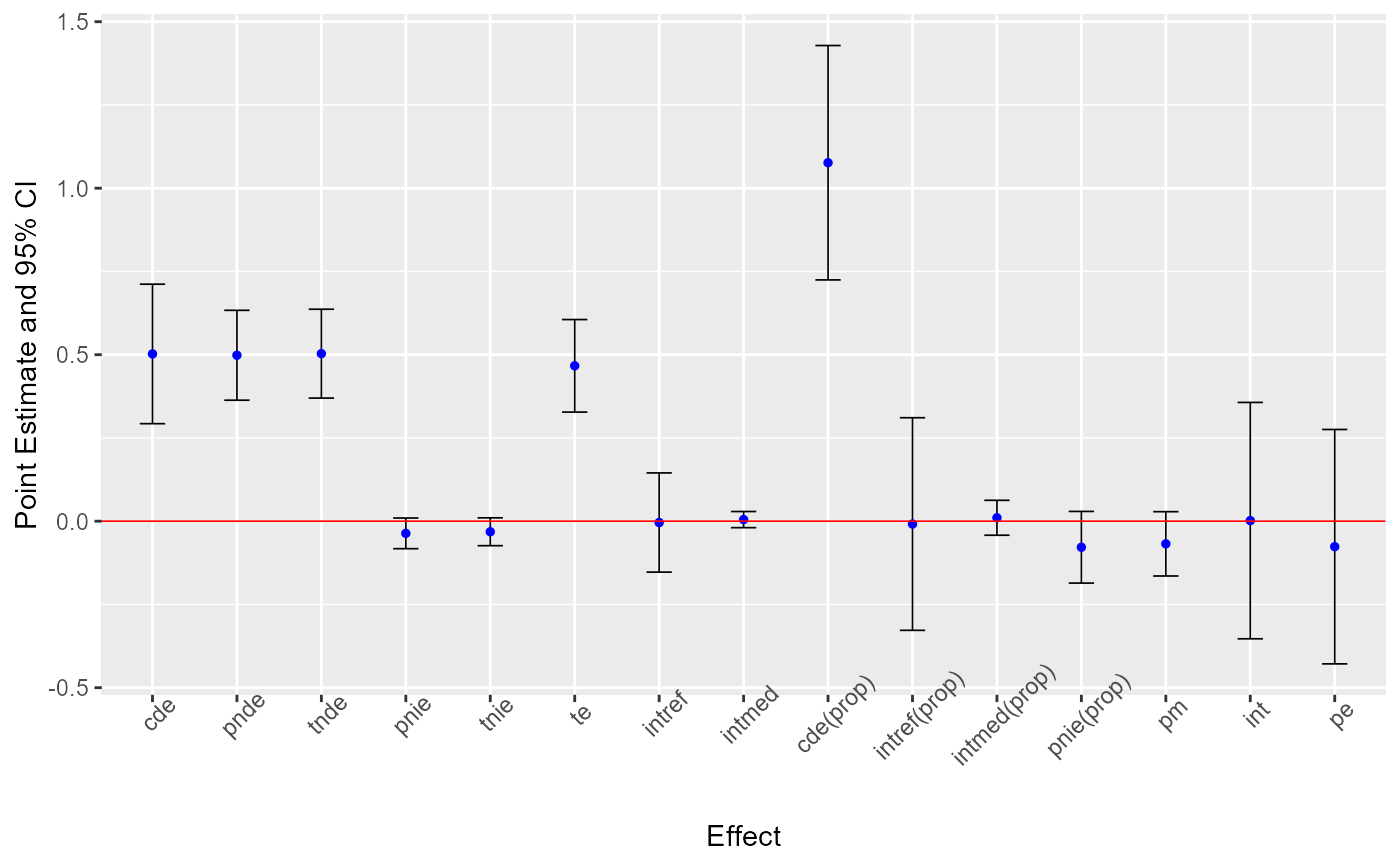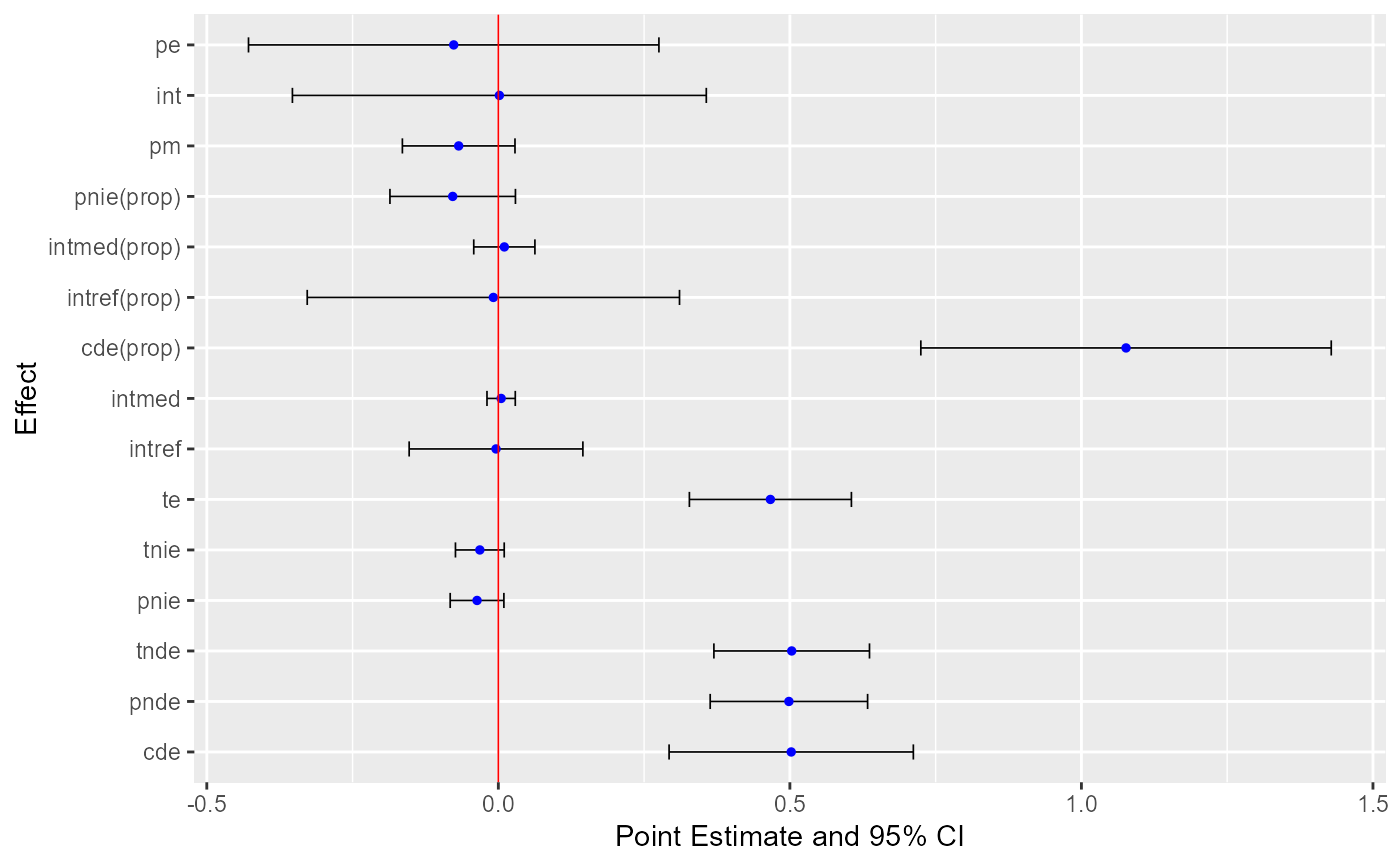ggcmest is used to plot results of cmest nicely with plotting functions in the ggplot2 package. Additional layers can be added to this plot using other plotting functions in the ggplot2 package.

ggcmest(
x,
errorbar.width = 0.3,
errorbar.size = 0.3,
errorbar.colour = "black",
point.size = 1,
point.colour = "blue",
refline = TRUE,
refline.colour = "red",
refline.size = 0.3
)

## Arguments

x an object of class cmest width of errorbars for confidence intervals. Default is 0.3. size of errorbars for confidence intervals. Default is 0.3. colour of errorbars for confidence intervals. Default is black. size of points for point estimates. Default is 1. colour of points for point estimates. Default is blue. a logical value. If true, include a reference line at y = 0 when effects are on the difference scale and include a reference line at y = 1 when effects are on the ratio scale. Default is TRUE. colour of the reference line. Default is red. size of the reference line. Default is 0.3.

cmest, ggplot2.

## Examples


library(CMAverse)
library(ggplot2)
#> Warning: package 'ggplot2' was built under R version 4.0.4
x <- cmest(data = cma2020, model = "rb", outcome = "contY",
exposure = "A", mediator = "M2", basec = c("C1", "C2"),
EMint = TRUE, mreg = list("multinomial"), yreg = "linear",
astar = 0, a = 1, mval = list("M2_0"), estimation = "paramfunc",
inference = "delta")

ggcmest(x) +
theme(axis.text.x = element_text(angle = 45))ggcmest(x) +
coord_flip(xlim = NULL, ylim = NULL, expand = TRUE, clip = "on")